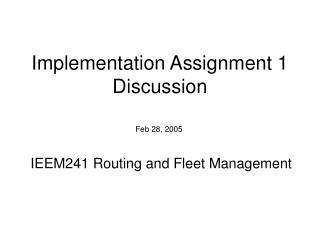# Implementation Assignment 1 Discussion - PowerPoint PPT PresentationDownload PresentationImplementation Assignment 1 Discussion

Implementation Assignment 1 Discussion
Download Presentation## Implementation Assignment 1 Discussion

- - - - - - - - - - - - - - - - - - - - - - - - - - - E N D - - - - - - - - - - - - - - - - - - - - - - - - - - -
##### Presentation Transcript

1. Implementation Assignment 1 Discussion Feb 28, 2005 IEEM241 Routing and Fleet Management

2. Outline • Complexity (all here are time complexity) • Review the examples in class • More examples • Implementation Assignment 1

4. Create object Example 1 • Add in the end of the list For each arc, searching end element of the link – O(n) Total m arcs, so the complexity is O(mn)

5. Example 1 • Add in the front of the list For each arc, create object in the front, point to exist first element – O(1) Total m arcs – O(m)

6. last_out_arc last_out_arc Example 1 – extension • Add in the end of the list With the help of last_out_arc, for each arc – O(1) Total m arcs – O(m)

7. Example 2 • Calculate Xn (X and n are integer) • Method 1: • Xn = X * X * X * … * X Total n X Complexity – O(n)

8. Example 2 • Method 2 • A0 = X • A1 = X2 = A0 * A0 • A2 = X4 = A1 * A1 • A3 = X8 = A2 * A2 • … • Ak = X2^k = Ak-1 * Ak-1, where 2k >= n/2 if ( 2k == n/2 ) Xn = 2Ak ; else { Y = 1; while ( n>0 ) { if ( n > 2k ) Y *= Ak; n = n – 2k; k – – ; } } O(lgn) O(lgn) Complexity – O(lgn)

9. Example 3 • Calculate a0 + a1*x + a2*x2 + … + an*xn ai , i = 0, 1, …, n and x are integer Method 1: each item, ai*xi = ai * x * x * … * x Total n items, the complexity is O(n2) O(n)

10. Example 3 • Method 2 a2x2+a1x+a0 = (a2*x+a1)*x + a0 a3x3+a2x2+a1x+a0= ((a3*x+a2)*x+a1)*x +a0 ...... an*xn + an-1*xn-1 + an-2*xn-2 + … + a1*x + a0 =(…((an*x + an-1)*x + an-2)*x + …a1)*x + a0 Complexity is O(n)

11. Conclusion • For the arc adding example • Usually, add in the front is more efficiency, O(m). • Without the pointer last_out_arc, add to the end cost O(mn);with the help of the pointer last_out_arc, the complexity of adding to the end is O(m), which indicates the importance of technical details in programming • All the three examples have shown the difference in time complexity between different algorithms

12. Implementation Assignment Discussion • Download t4-ia1.ppt(this slides) and the sample code ReadArc_t4.java and data file a1_data.txt from any of the mirror sites: • http://143.89.21.185/ieem241/t4/ • ftp://ieem241:ieem241@143.89.21.185/t4 • http://143.89.20.211/~kick/t4